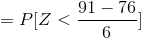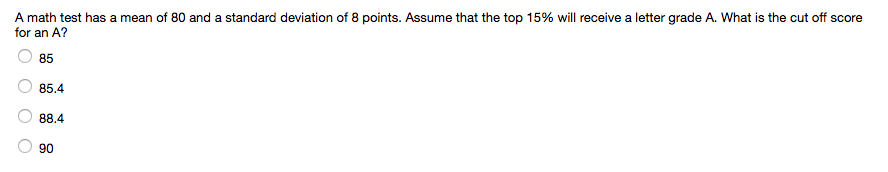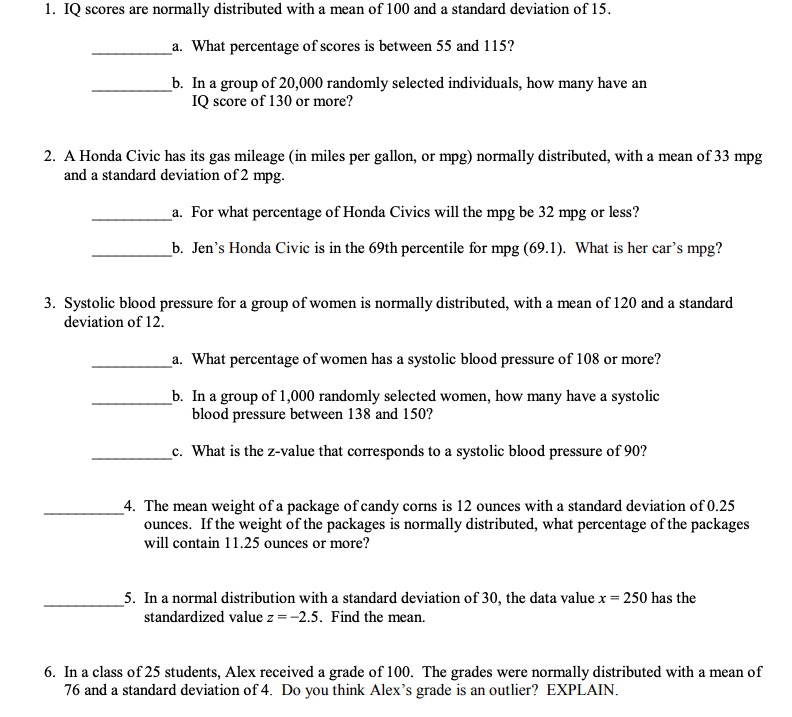Question

# a professor gives a test, the mean is 76 and standard deviation is 6 point. what...

a professor gives a test, the mean is 76 and standard deviation is 6 point. what is the percentage if the cut off mark is 91

P[X<91]=P[Z<2.5]

=0.9938....................by using Z table.

 Therefore, 99.38 %  if the cut off mark is 91

#### Earn Coins

Coins can be redeemed for fabulous gifts.

Similar Homework Help Questions
• ### A math test has a mean of 80 and a standard deviation of 8 points. Assume...A math test has a mean of 80 and a standard deviation of 8 points. Assume that the top 15% will receive a letter grade A. What is the cut off score for an A? 0 85 85.4 88.4 90

• ### The mean mark on a provincial standardized test is 66 with a standard deviation of 5,...

The mean mark on a provincial standardized test is 66 with a standard deviation of 5, following a normal distribution. What is the probability that a given student will not achieve a passing mark of at least 50?

• ### The algebra test had a mean of 82 and a standard deviation of 8. What percentage...

The algebra test had a mean of 82 and a standard deviation of 8. What percentage of scores fall between 82 and 90.

• ### Hugo averages 76 words per minute on a typing test with a standard deviation of 12.5...

Hugo averages 76 words per minute on a typing test with a standard deviation of 12.5 words per minute. Suppose Hugo's words per minute on a typing test are normally distributed. Let X= the number of words per minute on a typing test. Then, X∼N(76,12.5). Suppose Hugo types 66 words per minute in a typing test on Wednesday. The z-score when x=66 is ________. This z-score tells you that x=66 is ________ standard deviations to the ________ (right/left) of the...

• ### A test has a mean of 81 and a Standard Deviation of 8.  What percentage of cases...

A test has a mean of 81 and a Standard Deviation of 8.  What percentage of cases falls above a score of 77?   Below a score of 93?

• ### 1. IQ scores are normally distributed with a mean of 100 and a standard deviation of 15. a. What ...all questions. Do not round answers 1. IQ scores are normally distributed with a mean of 100 and a standard deviation of 15. a. What percentage of scores is between 55 and i 15? b. In a group of 20,000 randomly selected individuals, how many have an IQ score of 130 or more? 2. A Honda Civic has its gas mileage (in miles per gallon, or mpg) normally distributed, with a mean of 33 mpg and a standard deviation of...

• ### A normally distributed population has a mean of 76 and a standard deviation of 19. Determine...

A normally distributed population has a mean of 76 and a standard deviation of 19. Determine the probability that a random sample of size 22 has an average between 72 and 76. Round to four decimal places.

• ### Part A. Score on 25 point test is normally distributed with mean 22 and standard deviation...

Part A. Score on 25 point test is normally distributed with mean 22 and standard deviation 5.         You took a sample of 9 students. The mean for this group is 19.111111        Test the hypothesis that the performance of this group is different than the regular group. Use α=.05. What is the alternative hypothesis? What is the rejection region? Calculated z What is your decision? Yes or no? You believe that the group from an honors and would perform better than...

• ### 4. On standard IQ tests, the mean is 100 with standard deviation 15. The results are...

4. On standard IQ tests, the mean is 100 with standard deviation 15. The results are very close to fitting a normal curve. Suppose the IQ test is given to a very large group of people. Find the percentage of people whose IQ score is: a) Greater than 130 b) Less than 85 c) Between 85 and 115. d) To join Mensa, one must be in the 98th percentile among all people taking the IQ test. What is the cut...

• ### The mean and standard deviation of test scores are 80 and 5 respectively. Assuming a bell-shaped...

The mean and standard deviation of test scores are 80 and 5 respectively. Assuming a bell-shaped distribution, what percentage of test-takers scored above 90?